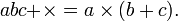# Polish notation

In mathematics and computer science, Polish notation is a way of expressing arithmetic or algebraic formulae which is unambiguous without the use of parentheses.

In ordinary "algebraic" or "infix" notation a binary operator such as × or + is written between the two operands, and an expression such as a × b + c is then ambiguous. One solution to this difficulty is to use a convention for priority or precedence, for example that multiplication precedes addition and then use brackets to show that the usual priority is not to be used (one such convention is "BODMAS"). Hence$(a \times b) + c = a \times b + c \neq a \times (b + c) . \,$

In prefix notation the operator precedes its two operands: the operand may be a term or another expression. So${+}{\times} a b c = {+} ({\times a b}) c = {+} (a \times b) c = (a \times b) + c \,;$${\times} a {+} b c = {\times} a ({+} b c) = {\times} a (b + c) = a \times (b + c) .\,$

Here brackets have been inserted to show the order in which the operations are performed, but are not part of or necessary for the notation.

In postifx or reverse Polish notation the operator follows its two operands.$a b {\times} c {+} = (a \times b) + c \,;$$a b c {+} {\times} = a \times (b + c) .\,$

Expressions in reverse Polish notation are particularly well adapted to evaluation on a stack.

The name "Polish notation" refers to the nationality of its inventor, Jan Łukasiewicz (1878-1956).

## Applications

Practical applications of Polish notations involve avoiding the need, and possible overhead, of parenthesizing to disambiguate the order of operations.

### Calculators

Hewlett-Packard scientific calculators traditionally use reverse Polish notation (RPN), while most other vendors used parenthesized algebraic notation. The human interface to a calculator does not necessarily show the expression being entered, so the human elegance of a parenthesized expression may not be available. Unquestionably, the learning curve for an RPN calculator is greater, but it allows fewer keystrokes.

### Programming languages

Various programming languages, often for resource constrained environments, such as FORTH, expect the original program to be written in a Polish notation. Other languages may not require Polish entry, but convert the program into an internal Polish form that efficiently runs on an interpreter.Some content on this page may previously have appeared on Citizendium.